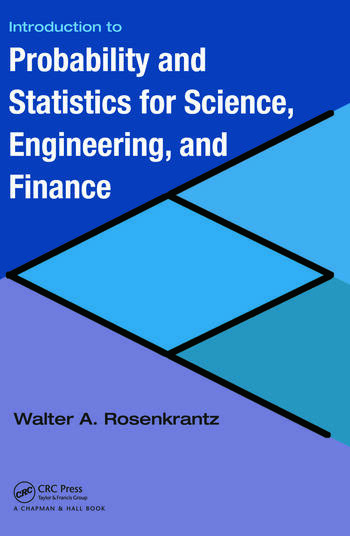# Introduction to Probability and Statistics for Science, Engineering, and Finance

## 1st Edition

Walter A. Rosenkrantz

Chapman and Hall/CRC
Published July 10, 2008
Textbook - 680 Pages - 99 B/W Illustrations
ISBN 9781584888123 - CAT# C8121

For Instructors Request Inspection Copy

was \$120.00

USD\$96.00

SAVE ~\$24.00

FREE Standard Shipping!

## Preview

### Summary

Integrating interesting and widely used concepts of financial engineering into traditional statistics courses, Introduction to Probability and Statistics for Science, Engineering, and Finance illustrates the role and scope of statistics and probability in various fields.

The text first introduces the basics needed to understand and create tables and graphs produced by standard statistical software packages, such as Minitab, SAS, and JMP. It then takes students through the traditional topics of a first course in statistics. Novel features include:

• Applications of standard statistical concepts and methods to the analysis and interpretation of financial data, such as risks and returns
• Cox–Ross–Rubinstein (CRR) model, also called the binomial lattice model, of stock price fluctuations
• An application of the central limit theorem to the CRR model that yields the lognormal distribution for stock prices and the famous Black–Scholes option pricing formula
• An introduction to modern portfolio theory
• Mean-standard deviation diagram of a collection of portfolios
• Computing a stock’s betavia simple linear regression
• As soon as he develops the statistical concepts, the author presents applications to engineering, such as queuing theory, reliability theory, and acceptance sampling; computer science; public health; and finance. Using both statistical software packages and scientific calculators, he reinforces fundamental concepts with numerous examples.

#### Instructors

We provide complimentary e-inspection copies of primary textbooks to instructors considering our books for course adoption.